Courses

# Lakhmir Singh & Manjit Kaur: Chemical Reactions and Equations, Solutions- 3 Class 10 Notes | EduRev

## Class 10 : Lakhmir Singh & Manjit Kaur: Chemical Reactions and Equations, Solutions- 3 Class 10 Notes | EduRev

The document Lakhmir Singh & Manjit Kaur: Chemical Reactions and Equations, Solutions- 3 Class 10 Notes | EduRev is a part of the Class 10 Course Class 10 Chemistry Solutions By Lakhmir Singh & Manjit Kaur.
All you need of Class 10 at this link: Class 10

(Page No - 23)

Question 50:
A silvery-white metal X taken in the form of ribbon, when ignited, burns in air with a dazzling white flame to form a white powder Y. When water is added to powder Y, it dissolves partially to form another substance Z.
(a) What could metal X be ?
(b) What is powder Y ?
(c) With which substance metal X combines to form powder Y ?
(d) What is substance Z ? Name one domestic use of substance Z.
(e) Write a balanced chemical equation of the reaction which takes place when metal X burns in air to form powder Y.
Solution :

(a) Magnesium, Mg.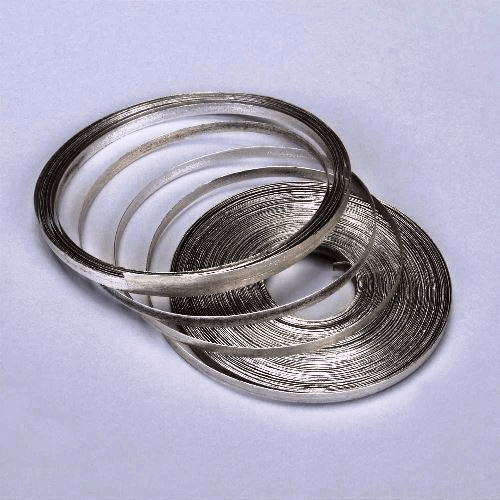Magnesium Ribbon(b) Magnesium oxide,MgO(c) Oxygen (of air),O2
(d) Magnesiumhydroxide, Mg(OH)2; Used as antacid to relieve indigestion
(e) 2Mg + O2 —–> 2MgO

Question 51:
A metal X forms a salt XSO4. The salt XSO4 forms a clear solution in water which reacts with sodium hydroxide solution to form a blue precipitate Y. Metal X is used in making electric wires and alloys like brass.
(a) What do you think metal X could be ?
(b) Write the name, formula and colour of salt XSO4.
(c) What is the blue precipitate Y ?
(d) Write a chemical equation of the reaction which takes place when salt XSO4 reacts with sodium hydroxide solution. Give the state symbols of all the reactants and products which occur in the above equation.
Solution :

(a) Copper, Cu.
(b) Copper sulphate, CuSO4, Blue colour.
(c) Copper hydroxide, Cu(OH)2
(d) CuSO(aq) + 2NaOH (aq) —–> Cu(OH)2 (s) + Na2S04 (aq)

Question 52:
The metal M reacts vigorously with water to form a solution S and a gas G. The solution S turns red litmus to blue whereas gas G, which is lighter than air, burns with a pop sound. Metal M has a low melting point and it is used as a coolant in nuclear reactors.
(a) What is metal M ?
(b) What is solution S ? Is it acidic or alkaline ?
(c) What is gas G ?
(d) Write a balanced chemical equation for the reaction which takes place when metal M reacts with water.
(e) Is this reaction exothermic or endothermic ?
Solution :

(a) Sodium, Na.
(b) Sodium hydroxide
solution (NaOH solution), Alkaline. (c) Hydrogen, H2
(d) 2Na + 2H2O —–> 2NaOH + H2
(e) Exothermic.

Question 53:
When a mixture of gases X and Y is compressed to 300 atm pressure and then passed over a catalyst consisting of a mixture of zinc oxide and chromium oxide (heated to a temperature of 300°C), then an organic compound Z having the molecular formula CH4O is formed. X is a highly poisonous gas which is formed in appreciable amounts when a fuel burns in a limited supply of air ; Y is a gas which can be made by the action of a dilute acid on an active metal; and Z is a liquid organic compound which can react with sodium metal to produce hydrogen gas.
(a) What are X, Y and Z ?
(b) Write a balanced chemical equation of the reaction which takes place when X and Y combine to form Z. Indicate the conditions under which the reaction occurs.
Solution :

(a) X is carbon monoxide gas (CO); Y is hydrogen gas (H2) ; Z is methanol (or Methyl alcohol) (CH3OH)
(CH40 = CH3OH)
(b) Formation of Z: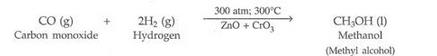The conditions for this reaction to take place are: a pressure of 300 atmospheres (written as 300 atm), a temperature of 300oC, and a catalyst which is a mixture of zinc oxide and chromium oxide (ZnO + CrO3).

Question 54:
The white solid compound A decomposes quite rapidly on heating in the presence of a black substance X to form a solid compound B and a gas C. When an aqueous solution of compound B is reacted with silver nitrate solution, then a white precipitate of silver chloride is obtained along with potassium nitrate solution. Gas C does not burn itself but helps burn other things.
(a) What is compound A ?
(b) What is compound B ?
(c) What is gas C ?
(d) What do you think is the black substance X ? What is its function ?
(e) What is the general name of substances like X ?
Solution :

(a) Potassium chlorate, KClO3
(b) Potassium chloride, KCl
(c) Oxygen, O2
(d) Manganese dioxide, MnO2; It acts as a catalyst in the decomposition of potassium chlorate to form oxygen gas
(e) Catalysts

Question 55:
Gas A, which is the major cause of global warming, combines with hydrogen oxide B in nature in the presence of an environmental factor C and a green material D to form a six carbon organic compound E and a gas F. The gas F is necessary for breathing.
(a) What is gas A ?
(b) What is the common name of B ?
(c) What do you think could be C ?
(d) What is material D ? Where is it found ?
Solution :

(a) Carbon dioxide,CO2
(b) Water, H2O
(c) Sunlight.
(d) Chlorophyll;Green leaves of plants.

(Page No - 45)

Question 1:
What type of reaction is represented by the digestion of food in our body ?
Solution :

Decomposition reaction.

Question 2:
Name the various types of chemical reactions.
Solution :

The various types of chemical reactions are:

1. Combination reactions.
2. Decomposition reaction.
3. Displacement reaction.
4. Double displacement reaction.
5. Oxidation and reduction reactions.

Question 3:
Why does-the colour of copper sulphate solution change when an iron nail is kept immersed in it ?
Solution :

The colour of copper sulphate solution changes when iron nail is kept immersed in it due to the displacement reaction taking place between iron and copper leading to formation of iron sulphate.

Question 4:
Write the balanced chemical equation for the following reaction :
Zinc + Silver nitrate —–> Zinc nitrate + Silver
Solution :

Zn + 2AgNO3 —–> Zn(NO3)2 + 2Ag

Question 5:
Which term is used to indicate the development of unpleasant smell and taste in fat and oil containing foods due to aerial oxidation (when they are kept exposed for a considerable time) ?
Solution :

Rancidity.

Question 6:
What is the general name of the chemicals which are added to fat and oil containing foods to prevent the development of rancidity
Solution :

Anti-oxidants.

Question 7:
State an important use of decomposition reactions.
Solution :

The digestion of food in the body is an example of decomposition reaction.

Question 8:
What are anti-oxidants ? Why are they added to fat and oil containing foods ?
Solution :

Anti-oxidant is a substance which prevents oxidation. They are added to fat and oil
containing foods so that they do not get oxidized easily and hence do not
turn rancid.

Question 9:
Explain why, food products containing fats and oils (like potato chips) are packaged in nitrogen.
Solution :

Food products containing fats and oils are packaged in nitrogen so that there is no oxygen to cause oxidation of food and make it rancid.

Question 10:
Give one example of a decomposition reaction which is carried out:
(a) with electricity
(b) by applying heat
Solution :

(a) When fused sodium chloride is decomposed by passing electricity, sodium metal is obtained.
(b) When lead nitrate is heated strongly, it breaks down to form lead monoxide, nitrogen dioxide and oxygen.

Question 11:
What type of chemical reaction is used to extract metals from their naturally occurring compounds like oxides or chlorides ?
Solution :

Decomposition
reactions (carried out by electricity).

Question 12:
Name two anti-oxidants which are usually added to fat and oil containing foods to prevent rancidity.
Solution :

BHA (Butylated
Hydroxy Anisole) and BHT (Butylated Hydroxy Toluene).

Question 13:
Write one equation each for the decomposition reactions where energy is supplied in the form of  (a) heat,
(b) light, and (c) electricity.
Solution :

(a) Decomposition reaction where heat is supplied for energy: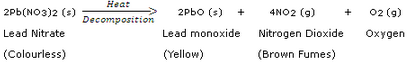(b) Decomposition reaction where light is supplied for energy: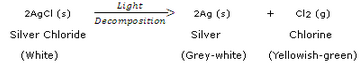(c) Decomposition reaction where electricity is supplied for energy: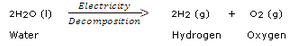Question 14:
In the refining of silver, the recovery of silver from silver nitrate solution involved displacement by copper metal. Write down the chemical equation of the reaction involved.
Solution :

2AgNO3 (aq) + Cu (s) —–>Cu(NO3)2 (aq) + 2Ag (s)

Question 15:
What type of reactions are represented by the following equations ?

1. CaC03 ——- > CaO + C02
2. CaO + H20 ——- > Ca(OH)2
3. 2FeSO4 ——- >Fe203 + S0+ S03
4. NH4Cl  —–> NH3+HCl
5. 2Ca+O2 ——- > 2CaO

Solution :

1. Decomposition.
2. Combination.
3. Decomposition.
4. Decomposition.
5. Combination.

(Page No - 46)

Question 16:
What type of chemical reactions take place when :
(a) a magnesium wire is burnt in air ?
(b) lime-stone is heated ?
(c) silver bromide is exposed to sunlight ?
(d) electricity is passed through water ?
(e) ammonia and hydrogen chloride are mixed ?
Solution :

(a) Combination.
(b) Decomposition.
(c) Decomposition.
(d) Decomposition.
(e) Combination.

Question 17:
What type of chemical reactions are represented by the following equations ?

1. A + BC ——- > AC + B
2. A + B ——- > C
3. X ——- > Y + Z
4. PQ + RS —— > PS + RQ
5. A2O3 + 2B —–> B2O3 + 2A

Solution :

1. Displacement reaction.
2. Combination reaction.
3. Decomposition reaction.
4. Double displacement reaction.
5. Displacement reaction.

Question 18:
Balance the following chemical equations :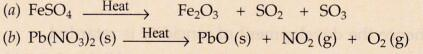Solution :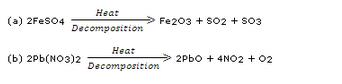Question 19:
Which of the following is a combination and which is a displacement reaction ?
(a) Cl2 + 2KI ——-> 2KCl + I2
(b) 2K + Cl2 ——–> 2KCl
Solution :

(a) Displacement reaction.
(b) Combination reaction.

Question 20:
What type of reactions are represented by the following equations ?
(a) CaO + CO2 ———- > CaCO3
(b) 2Na + 2H2O —— > 2NaOH + H2
(c) Mg + CuS04——— > MgSO+ Cu
(d) NH4NO2 ——- > N2 + 2H2O
(e) CuS04 + 2NaOH ——– > Cu(OH)2 + Na2SO4
Solution :

(a) Combination reaction.
(b) Displacement reaction.
(c) Displacement reaction.
(d) Decomposition reaction.
(e) Double displacement reaction.

Question 21:
In the following reaction between lead sulphide and hydrogen peroxide :
PbS (s) + 4H2O(aq) ————-> PbS04 (s) + 4H2O (l)
(a) Which substance is reduced ?
(b) Which substance is oxidised ?
Solution :

(a) H2O2
(b) PbS

Question 22:
Identify the component oxidised in the following reaction :
H2S + Cl2 ————->S + 2HCl
Solution :

H2S

Question 23:
When S02 gas is passed through saturated solution of H2S, the following reaction occurs :
SO2 + 2H2S ———– > 2H2O + 3S
In this reaction, which substance is oxidised and which one is reduced ?
Solution :

Substance oxidised: H2S
Substance reduced: SO2

Question 24:
Fill in the following blanks with suitable words :
(a) The addition of oxygen to a substance is called ……………………… whereas removal of oxygen is
called……
(b) The addition of hydrogen to a substance is called……… whereas removal of hydrogen is called………..
(c) Anti-oxidants are often added to fat containing foods to prevent………….. due to oxidation.
Solution :

(a) Oxidation; reduction.
(b) Reduction; oxidation.
(c) Rancidity.

Question 25:
What is an oxidation reaction ? Identify in the following reaction
(i) the substance oxidised, and (ii) the substance reduced :
ZnO + C ———— > Zn + CO
Solution :

Oxidation Reaction: The addition of oxygen (or removal of hydrogen) to a substance is called oxidation.
(i) C (ii) ZnO

Question 26:
(a) What is a redox reaction ? Explain with an example.
(b) When a magnesium ribbon burns in air with a dazzling flame and forms a white ash, is magnesium oxidised or reduced ? Why ?
(c) In the reaction represented by the equation :
MnO2 + 4Hl ———–> MnCl2 + 2H2O + Cl2

1. name the substance oxidised.
2. name the oxidising agent.
3. name the substance reduced.
4. name the reducing agent.

Solution :
(a) The oxidation and reduction reactions occurring together are called a redox reaction. Example:
In this reaction, copper oxide is being reduced to copper whereas hydrogen is being oxidised to water.
(b) Magnesium is oxidised as addition of oxygen to magnesium takes place leading to formation of magnesium oxide.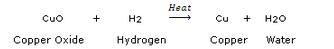(c)

1. HCl
2. MnO2
3. MnO2
4.  HCl

(Page No - 47)

Question 27:
(a) Define a combination reaction.
(b) Give one example of a combination reaction that is also exothermic.
(c) Give one example of a combination reaction that is also endothermic.
Solution :

(a) Those reactions in which two or more substances combine to form a single substance is called
a combination reaction.

Question 28:
(a) Give an example of an oxidation reaction.
(b) Is oxidation an exothermic or an endothermic reaction?
(c) Explain, by giving an example, how oxidation and reduction proceed side by side.
Solution :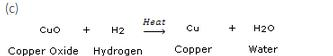In this reaction, copper oxide is being reduced to copper whereas hydrogen is being oxidised to water.

Question 29:
(a) What is the colour of ferrous sulphate crystals ? How does this colour change   after heating ?
(b) Name the product formed on strongly heating ferrous sulphate crystals. What type of chemical reaction
occurs in this change ?
Solution :

(a) The colour of ferrous sulphate is green. It changes to brown after heating.
(b) The product formed is ferric oxide. This is a decomposition reaction.

Question 30:
What is a decomposition reaction ? Give an example of a decomposition reaction. Describe an activity to illustrate such a reaction by heating.
Solution :

Those reactions in which a compound splits up into tvvo or more simpler substances is called a decomposition reaction.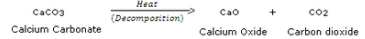When calcium carbonate is heated, it decomposes to give calcium oxide and carbon dioxide.
Activity: When potassium chlorrate is heated in the presence of manganese dioxide catalyst, it decomposes to give potassium chloride and Oxygen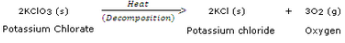This decomposition takes place in the presence of heat and catalyst. In this decomposition reaction, a single compound, potassium chlorate, is splitting up into two simpler substances, potassium chloride and oxygen. This decomposition reaction is used for preparing oxygen gas in the laboratory.

Question 31:
Zinc oxide reacts with carbon, on heating, to form zinc metal and carbon monoxide. Write a balanced chemical equation for this reaction. Name (i) oxidising agent, and (ii) reducing agent, in this reaction.
Solution :

ZnO + C
(i) Zinc oxide.
(ii) Carbon.

Question 32:
Give one example of an oxidation-reduction reaction which is also :
(a) a combination reaction
(b) a displacement reaction
Solution :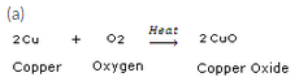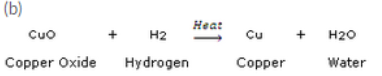Question 33:
(a) What is the difference between displacement and double displacement reactions ? Write equations for
these reactions.
(b) What do you mean by a precipitation reaction ? Explain giving an example.
Solution :

(a) Those reactions in which one element takes the place of another element in a compound, are known as displacement reactions.
Equation: CuSO4 (aq)+ Zn (s)—–>ZnSO4+ Cu
Those reactions in which two compounds react by an exchange of ions to form two new compounds are called double displacement reactions.
Equation: AgNO3 (aq) + NaCl (aq)—–>AgCl (s)+ NaNO3 (aq)
(b) Any reaction in which an insoluble solid (called precipitate) is formed that separates from the solution is called a precipitation reaction.
Example: The reaction between barium chloride and sodium sulphate solution to form a white barium sulphate precipitate (alongwith sodium chloride solution) is an example of a precipitation reaction.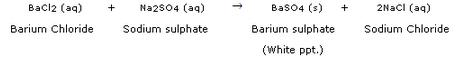Question 35:
(a) Define the following in terms of gain or loss of hydrogen with one example each :
(i) oxidation (ii) reduction
(b) When a magnesium ribbon is heated, it burns in air to form magnesium oxide. Write a balanced chemical equation for this reaction. Name (i) substance oxidised, and (ii) substance reduced.
Solution :

(a) (i) H2S + Cl2—–>S + 2HCl
In this reaction, H2S is changing into S. That is, Hydrogen is being removed from hydrogen sulphide. Now, by definition, the removal of hydrogen from a compound is called oxidation, so, we can say that hydrogen sulphide is being oxidised to sulphur. On the other hand, Cl2 is changing into HCl. That is, hydrogen is being added to chlorine. By definition, the addition of hydrogen to a substance is called reduction, so we can say that chlorine is being reduced to hydrogen chloride.
(b) 2Mg + O2—–>2MgO
(i) Mg (ii) O2

Question 36:
What is meant by (a) displacement reaction, and (b) double displacement reaction ? Explain with the help of one example each.
Solution :

(a) Those reactions in which one element takes the place of another element in a compound, are known as displacement reactions.
Example: CuSO4 (aq) + Zn (s) —–>ZnSO4 + Cu
When a strip of zinc metal is placed in copper sulphate solution, then zinc sulphate solution and copper are obtained. In this reaction, zinc displaces copper from copper sulphate compound so that copper is set free. The blue colour of copper sulphate solution fades due to the formation of zinc sulphate.
(b) Those reactions in which two compounds react by an exchange of ions to form two new compounds are called double displacement reactions.
Example: AgNO3 (aq) + NaCl (aq)
When silver nitrate solution is added to sodium chloride solution, then a white precipitate of silver chloride is formed along with sodium nitrate solution. In this reaction, two compounds, silver nitrate and sodium chloride, react to form two new compounds, silver chloride and sodium nitrate.

Question 37:
(a) Why are decomposition reactions called the opposite of combination reactions ? Explain with equations
of these reactions.
(b) Express the following facts in the form of a balanced chemical equation :
“When a strip of copper metal is placed in a solution of silver nitrate, metallic silver is precipitated and a solution containing copper nitrate is formed”.
Solution :

Those reactions in which a compound splits up into two or more simpler substances are known as decomposition reactions.
Example: When calcium carbonate is heated, it decomposes to give calcium oxide and carbon dioxide.
They are called opposite of combination reactions because in a combination reaction, two or more substances combine to form a single substance.
Example: Magnesium and oxygen combine, when heated, to form magnesium oxide.
(b) Cu (s) + 2AgNO3 (aq)—–>Cu(NO3)2 (aq) + 2Ag (s)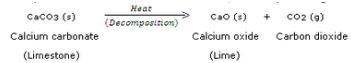They are called opposite of combination reactions because in a combination reaction, two or more substances combine to form a single substance.

Example: Magnesium and oxygen combine, when heated, to form magnesium oxide.

2Mg (s) Magnesium + 02 oxygen → 2Mg0 (s) Magnesium Oxide

Question 38:
(a) What happens when a piece of iron metal is placed in copper sulphate solution ? Name the type ofreaction involved.
(b) Write balanced chemical equation with state symbols for the following reaction :Barium chloride solution reacts with sodium sulphate solution to give insoluble barium sulphate and a solution of sodium chloride.
Solution :

(a) When a piece of iron metal is placed in copper sulphate solution, then magnesium sulphate solution and copper metal are formed. This is a type of displacement reaction.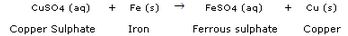(b)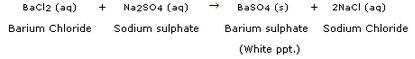Question 39:
In the reaction represented by the following equation :
CuO(s) + H2(g) ————- > Cu (s) + H20(1)
(a) name the substance oxidised
(b) name the substance reduced
(c) name the oxidising agent
(d) name the reducing agent
Solution :

(a) H2
(b) CuO
(c) CuO
(d) H2

Question 40:
What happens when silver nitrate solution is added to sodium chloride solution ?
(a) Write the equation for the reaction which takes place.
(b) Name the type of reaction involved.
Solution :

When silver nitrate
solution is added to sodium chloride solution, then a white precipitate of
silver chloride is formed alongwith sodium nitrate solution.
(a) AgNO3(aq) + NaCl (aq)
(b) Double displacement reaction.

Question 41:
What happens when silver chloride is exposed to sunlight ? Write a chemical equation for this reaction. Also give one use of such a reaction.
Solution :

When silver chloride is exposed to light, it decomposes to form silver metal and chlorine gas.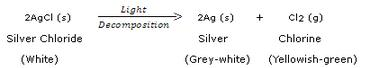This reaction is used in black and white photography.

Question 42:
What happens when a zinc strip is dipped into a copper sulphate solution ?
(a) Write the equation for the reaction that takes place.
(b) Name the type of reaction involved.
Solution :

When a strip of zinc metal is placed in copper sulphate solution, then zinc sulphate solution and copper are obtained.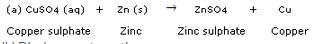(Page No - 48)

Question 43:
(a) Explain the term “corrosion” with an example. Write a chemical equation to show the process of corrosion of iron.
(b) What special name is given to the corrosion of iron ?
(c) What type of chemical reaction is involved in the corrosion of iron ?
(d) Name any three objects (or structures) which are gradually damaged by the corrosion of iron and steel.
Solution :

(a) Corrosion is the process in which metals are eaten up gradually by the action of air, moisture or a chemical (such as an acid) on their surface. Rusting of iron metal is the most common form of corrosion.
During the corrosion of iron, iron metal is oxidised by the oxygen of air in the presence of water (moisture) to form hydrated iron oxide called rust.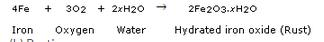(b) Rusting.
(c) Oxidation.
(d) Railings, car bodies and bridges.

Question 44:
(a) Explain the term “rancidity”. What damage is caused by rancidity ?
(b) What type of chemical reaction is responsible for causing rancidity ?
(c) State and explain the various methods for preventing or retarding rancidity of food.
Solution :

(a) The condition produced by aerial oxidation of fats and oils in foods marked by unpleasant smell and taste is called rancidity. It spoils the food materials prepared in fats and oils which have been kept for a considerable time and makes them unfit for eating.
(b) Oxidation.
(c) Rancidity can be prevented by adding anti-oxidants to foods containing fats and oils.

1. It can be prevented by packaging fat and oil containing foods in nitrogen gas.
2. Rancidity can be retarded by keeping food in a refrigerator.
3. Rancidity can be prevented by storing food in air-tight containers.
4. Storing foods away from light can also prevent rancidity.

Question 45:
(a) What happens when an aqueous solution of sodium sulphate reacts with an aqueous solution of barium chloride ?
(b) Write the balanced chemical equation for the reaction which takes place.
(c) State the physical conditions of reactants in which the reaction will not take place.
(d) Name the type of chemical reaction which occurs.
(e) Give one example of another reaction which is of the same type as the above reaction.
Solution :

(a) When barium chloride solution is added to sodium sulphate solution, then a white precipitate of barium sulphate is formed along with sodium chloride solution.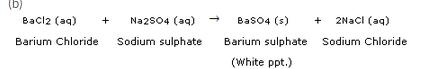(c) Solid sodium sulphate and Solid barium chloride
(d) Double displacement reaction.
(e) Double displacement reaction between silver nitrate solution and sodium chloride solution forms a white precipitate of silver chloride and sodium nitrate solution.

(Page No - 49)

Question 58:
When a green iron salt is heated strongly, its colour finally changes to brown and odour of burning sulphur is given out.
(a) Name the iron salt.
(b) Name the type of reaction that takes place during the heating of iron salt.
(c) Write a chemical equation for the reaction involved.
Solution :

(a) Ferrous sulphate.
(b) Decomposition reaction.
(c)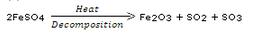Question 59:
A colourless lead salt, when heated, produces a yellow residue and brown fumes.
(b) Name the brown fumes.
(c) Write a chemical equation of the reaction involved.
Solution :

(b) Nitrogen dioxide.
(c)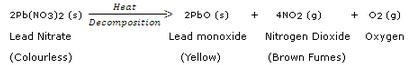Question 60:
When hydrogen burns in oxygen, water is formed and when water is electrolysed, then hydrogen and oxygen are produced. What type of reaction takes place :
(a) in the first case ?
(b) in the second case ?
Solution :

(a) Combination reaction.
(b) Decomposition reaction.

Question 61:
A strip of metal X is dipped in a blue coloured salt solution YSO4. After some time, a layer of metal Y from the salt solution is formed on the surface of metal strip X. Metal X is used in galvanisation whereas metal Y is used in making electric wires. Metal X and metal Y together form an alloy Z.
(a) What could metal X be ?
(b) What could metal Y be ?
(c) Name the metal salt YSO4.
(d) What type of chemical reaction takes place when metal X reacts with salt solution YSO4 ? Write the equation of the chemical reaction involved.
(e) Name the alloy Z.
Solution :

(b) Copper (Cu)
(c) Copper sulphate (CuSO4)
(d) Displacement reaction;
CuSO(aq) + Zn (s)
ZnSO4 (aq) + Cu (s)
(e) Brass

Question 62:
When a black metal compound XO is heated with a colourless gas Y2, then metal X and another compound Y2O are formed. Metal X is red-brown in colour which does not react with dilute acids at all. Gas Y2 can be prepared by the action of a dilute acid on any active metal. The compound Y2O is a liquid at room temperature which can turn anhydrous copper sulphate blue.
(a) What do you think is metal X ?
(b) What could be gas Y2 ?
(c) What is compound XO ?
(d) What is compound Y2O?
(e) Write the chemical equation of the reaction which takes place on heating XO with Y2.
(f) What type of chemical reaction is illustrated in the above equation ?
Solution :

(a) Copper (Cu)
(b) Hydrogen (H2)
(c) Copper oxide (CuO)
(d) Water (H2O)
(e) CuO + H2
Cu + H2O
(f) Displacement reaction (which is also a redox reaction).

Question 63:
A metal X forms a water soluble salt XNO3 . When an aqueous solution of XNO3 is added to common salt solution, then a white precipitate of compound Y is formed along with sodium nitrate solution. Metal X is said to be the best conductor of electricity and it does not evolve hydrogen when put in dilute hydrohloric acid.
(a) What is metal X ?
(b) What is salt XNO3 ?
(c) Name the compound Y.
(d) Write the chemical equation of the reaction which takes place on reacting XNO3 solution and common salt solution giving the physical states of all the reactants and products.
(e) What type of chemical reaction is illustrated by the above equation ?
Solution :

Silver (Ag)
(b) Silver nitrate (AgNO3)
(c) Silver chloride (AgCl)
(d) AgNO3(aq) + NaCl (aq)—–>AgCl (s) + NaNO3 (aq)
(e) Double displacement reaction.

(Page No - 50)

Question 64:
Two metals X and Y form the salts XSO4 and Y2SO4, respectively. The solution of salt XSO4 is blue in colour whereas that of Y2SO4 is colourless. When barium chloride solution is added to XSO4 solution, then a white precipitate Z is formed along with a salt which turns the solution green. And when barium chloride solution is added to Y2SO4 solution, then the same white precipitate Z is formed along with colourless common salt solution.
(a) What could the metals X and Y be ?
(b) Write the name and formula of salt XSO4.
(c) Write the name and formula of salt Y2SO4.
(d) What is the name and formula of white precipitate Z ?
(e) Write the name and formula of the salt which turns the solution green in the first case.
Solution :

(a) Metal X : Copper; Metal Y : Sodium
(b) Copper sulphate, CuSO4
(c) Sodium sulphate, Na2SO4
(d) Barium sulphate, BaSO4
(e) Copper chloride, CuCl2

Question 65:
A red-brown metal X forms a salt XSO4. When hydrogen sulphide gas is passed through an aqueous solution of XSO4, then a black precipitate of XS is formed alongwith sulphuric acid solution.
(a) What could the salt XSO4 be ?
(b) What is the colour of salt XSO4 ?
(c) Name the black precipitate XS.
(d) By using the formula of the salt obtained in (a) above, write an equation of the reaction which takes place when hydrogen sulphide gas is passed through its aqueous solution.
(e) What type of chemical reaction takes place in this case ?
Solution :

(a) Copper sulphate.
(b) Blue colour.
(c) Copper sulphide.
(d) CuSO4 (aq) + H2S (g)—–>CuS (s) + H2SO(aq)
(e) Double displacement reaction.

Question 66:
When a strip of red-brown metal X is placed in a colourless salt solution YNOthen metal Y is set free and a blue coloured salt solution X(NO3)2 is formed. The liberated metal Y forms a shining white deposit on the strip of metal X.
(a) What do you think metal X is ?
(b) Name the salt YNO3.
(c) What could be metal Y ?
(d) Name the salt X(NO3)2.
(e) What type of reaction takes place between metal X and salt solution YNO3 ?
Solution :

(a) Copper.
(b) Silver nitrate.
(c) Silver.
(d) Copper nitrate.
(e) Displacement reaction.

Question 67:
A metal salt MX when exposed to light splits up to form metal M and a gas X2. Metal M is used in making ornaments whereas gas X2 is used in making bleaching powder. The salt MX is itself used in black and white photography.
(a) What do you think metal M is ?
(b) What could be gas X2 ?
(c) Name the metal salt MX.
(d) Name any two salt solutions which on mixing together can produce a precipitate of salt MX.
(e) What type of chemical reaction takes place when salt MX is exposed to light ? Write the equation of the reaction.
Solution :

(a) Silver.
(b) Chlorine.
(c) Silver chloride.
(d) Silver nitrate and Sodium chloride.
(e) Decomposition reaction;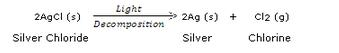Offer running on EduRev: Apply code STAYHOME200 to get INR 200 off on our premium plan EduRev Infinity!

20 docs

,

,

,

,

,

,

,

,

,

,

,

,

,

,

,

,

,

,

,

,

,

,

,

,

;